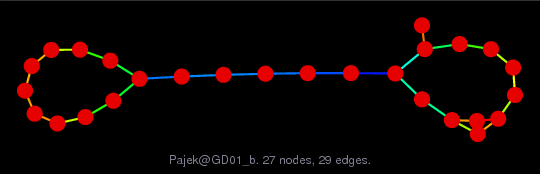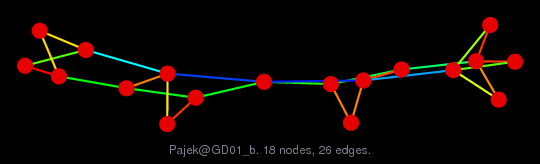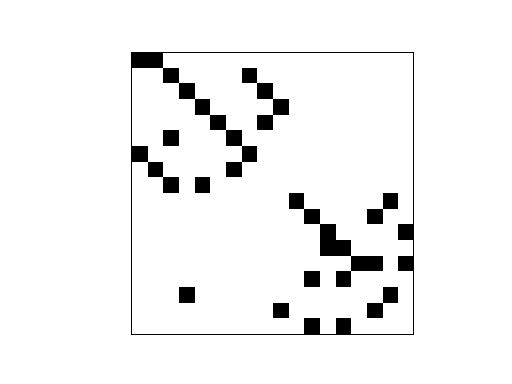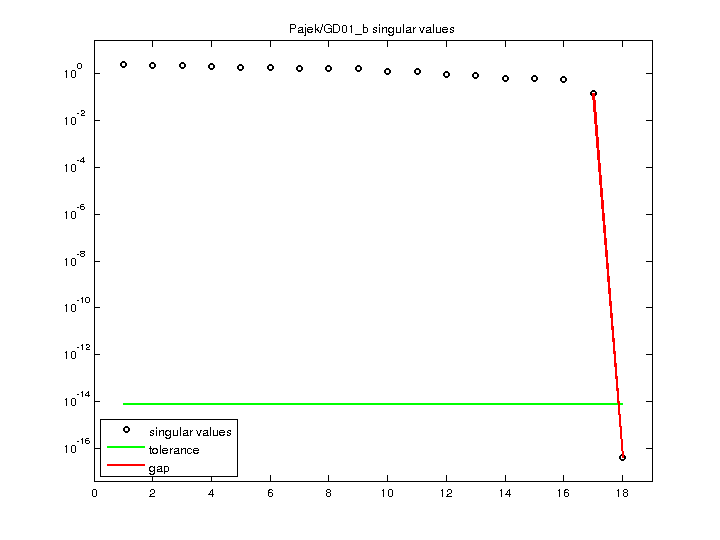Matrix: Pajek/GD01_b

Description: Pajek network: Graph Drawing contest 2001(bipartite graph drawing) (graph drawing of A+A')• Matrix group: Pajek
• download as a MATLAB mat-file, file size: 1 KB. Use UFget(1479) or UFget('Pajek/GD01_b') in MATLAB.

 Matrix properties number of rows 18 number of columns 18 nonzeros 37 # strongly connected comp. 1 explicit zero entries 0 nonzero pattern symmetry 51% numeric value symmetry 51% type binary structure unsymmetric Cholesky candidate? no positive definite? no

 author Graph Drawing Contest editor V. Batagelj date 2001 kind directed graph 2D/3D problem? no

 Additional fields size and type nodename full 18-by-22 coord full 18-by-2

Notes:

```------------------------------------------------------------------------------
Pajek network converted to sparse adjacency matrix for inclusion in UF sparse
matrix collection, Tim Davis.  For Pajek datasets, See V. Batagelj & A. Mrvar,
------------------------------------------------------------------------------
The original problem had 3D xyz coordinates, but all values of z were equal
to 0.5, and have been removed.  This graph has 2D coordinates.
```

 SVD-based statistics: norm(A) 2.35797 min(svd(A)) 4.25605e-17 cond(A) 5.54028e+16 rank(A) 17 null space dimension 1 full numerical rank? no singular value gap 3.29294e+15

 singular values (MAT file): click here SVD method used: s = svd (full (A)) status: ok